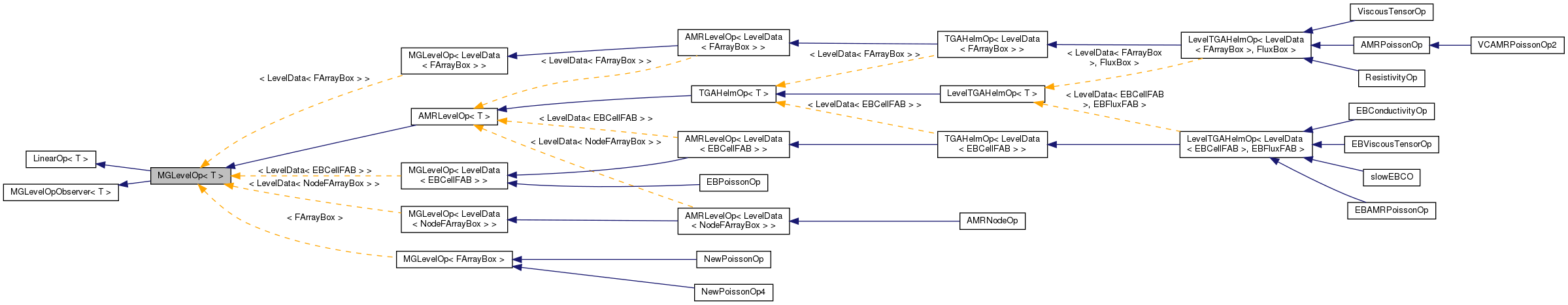# MGLevelOp< T > Class Template Reference

`#include <MultiGrid.H>`

Inheritance diagram for MGLevelOp< T >:[legend]

## Detailed Description

### template<typename T> class MGLevelOp< T >

This class handles the additional tasks of coordinating operations between this level and the level one coarser than this 'level'. This class is also an MGLevelOpObserver, so objects of this type can observe other MGLevelOp objects.

## Public Member Functions

MGLevelOp ()
Constructor.
virtual ~MGLevelOp ()
Destructor.
virtual void createCoarser (T &a_coarse, const T &a_fine, bool ghosted)=0
virtual void relax (T &a_correction, const T &a_residual, int a_iterations)=0
virtual void restrictResidual (T &a_resCoarse, T &a_phiFine, const T &a_rhsFine)=0
virtual void prolongIncrement (T &a_phiThisLevel, const T &a_correctCoarse)=0
void addObserver (MGLevelOpObserver< T > *a_observer)
void removeObserver (MGLevelOpObserver< T > *a_observer)
void addCoarserObserver (MGLevelOp< T > *a_operator, int a_coarseningFactor)
void notifyObserversOfChange ()
This should be called whenever the operator's data is updated.
virtual void finerOperatorChanged (const MGLevelOp< T > &a_operator, int a_coarseningFactor)
int numObservers () const
Returns the number of objects observing this operator.

## Private Member Functions

MGLevelOp (const MGLevelOp &)
MGLevelOpoperator= (const MGLevelOp &)

## Private Attributes

std::vector< MGLevelOpObserver
< T > * >
m_observers
List of observers for the operator.
std::vector< int > m_coarseningFactors
Multigrid coarsening factors.

## Constructor & Destructor Documentation

template<typename T>
 MGLevelOp< T >::MGLevelOp ( ) ` [inline]`

Constructor.

template<typename T>
 virtual MGLevelOp< T >::~MGLevelOp ( ) ` [inline, virtual]`

Destructor.

template<typename T>
 MGLevelOp< T >::MGLevelOp ( const MGLevelOp< T > & ) ` [private]`

## Member Function Documentation

template<typename T>
 virtual void MGLevelOp< T >::createCoarser ( T & a_coarse, const T & a_fine, bool ghosted ) ` [pure virtual]`

Create a coarsened (by two) version of the input data. This does not include averaging the data. So if a_fine is over a Box of (0, 0, 0) (63, 63, 63), a_fine should be over a Box (0, 0, 0) (31, 31, 31).

template<typename T>
 virtual void MGLevelOp< T >::relax ( T & a_correction, const T & a_residual, int a_iterations ) ` [pure virtual]`

Use your relaxtion operator to remove the high frequency wave numbers from the correction so that it may be averaged to a coarser refinement. A point relaxtion scheme, for example takes the form a_correction -= lambda*(L(a_correction) - a_residual).

template<typename T>
 virtual void MGLevelOp< T >::restrictResidual ( T & a_resCoarse, T & a_phiFine, const T & a_rhsFine ) ` [pure virtual]`

calculate restricted residual a_resCoarse[2h] = I[h->2h] (rhsFine[h] - L[h](phiFine[h])

template<typename T>
 virtual void MGLevelOp< T >::prolongIncrement ( T & a_phiThisLevel, const T & a_correctCoarse ) ` [pure virtual]`

correct the fine solution based on coarse correction a_phiThisLevel += I[2h->h](a_correctCoarse)

template<typename T>
 void MGLevelOp< T >::addObserver ( MGLevelOpObserver< T > * a_observer ) ` [inline]`

This adds a new observer to this operator. Note that this operator does not own the resources for the observer, so you must be careful to ensure that the observer does not go out of scope while the operator lives. If the observer is already observing the operator, this operation has no effect.

Parameters:
 a_observer An observer to be notified when the operator changes.

template<typename T>
 void MGLevelOp< T >::removeObserver ( MGLevelOpObserver< T > * a_observer ) ` [inline]`

Removes the given observer from the operator's list of observers. This method has no effect if the observer is not found in the list.

Parameters:
 a_observer An observer to be removed from the operator's observer list.

template<typename T>
 void MGLevelOp< T >::addCoarserObserver ( MGLevelOp< T > * a_operator, int a_coarseningFactor ) ` [inline]`

Adds a MGLevelOp observer to this operator that is notified when the operator changes so that it can coarsen its corresponding data. This is used to implement dependencies between multigrid operators at different grid levels.

Parameters:
 a_operator The multigrid operator that will observe this operator. No operator may observe itself. If a_operator is already observing this operator, this method has no effect. a_coarseningFactor The refinement factor between the grid level for a_operator and that for this one.

template<typename T>
 void MGLevelOp< T >::notifyObserversOfChange ( ) ` [inline]`

This should be called whenever the operator's data is updated.

Notify our observers that the operator's data has changed, taking special care to pass multigrid operators their level.

template<typename T>
 virtual void MGLevelOp< T >::finerOperatorChanged ( const MGLevelOp< T > & a_operator, int a_coarseningFactor ) ` [inline, virtual]`

Overload this method to implement the response of a multigrid operator to a change in a finer operator that it is observing. This allows the coarser observer to coarsen any data that has been changed in the finer operator.

Parameters:
 a_operator The operator whose state has changed. a_coarseningFactor The factor by which this operator's data is coarsened with respect to that of a_operator.

Reimplemented in VCAMRPoissonOp2, EBConductivityOp, and EBViscousTensorOp.

template<typename T>
 int MGLevelOp< T >::numObservers ( ) const` [inline]`

Returns the number of objects observing this operator.

template<typename T>
 MGLevelOp& MGLevelOp< T >::operator= ( const MGLevelOp< T > & ) ` [private]`

## Member Data Documentation

template<typename T>
 std::vector*> MGLevelOp< T >::m_observers` [private]`

template<typename T>
 std::vector MGLevelOp< T >::m_coarseningFactors` [private]`

The documentation for this class was generated from the following file:

Generated on Tue Mar 19 03:43:18 2019 for Chombo + EB by1.5.5